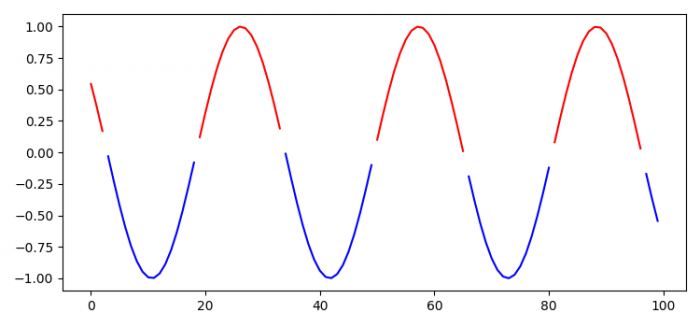# Plot a multicolored line based on a condition in Python Matplotlib

To plot a multicolored line based on a condition in Python Matplotlib, we can take the following steps −

• Set the figure size and adjust the padding between and around the subplots.

• Create y data points using numpy.

• Make l and u data points to differentiate the colors.

• Plot the u and l data points using plot() method, with different colors.

• To display the figure, use show() method.

## Example

import matplotlib.pyplot as plt
import numpy as np

plt.rcParams["figure.figsize"] = [7.50, 3.50]
plt.rcParams["figure.autolayout"] = True

y = np.sin(np.linspace(-10, 10, 100))

u = y.copy()
l = y.copy()

u[u <= 0] = np.nan
l[l >= 0] = np.nan

plt.plot(u, color='red')
plt.plot(l, color='blue')

plt.show()

## Output